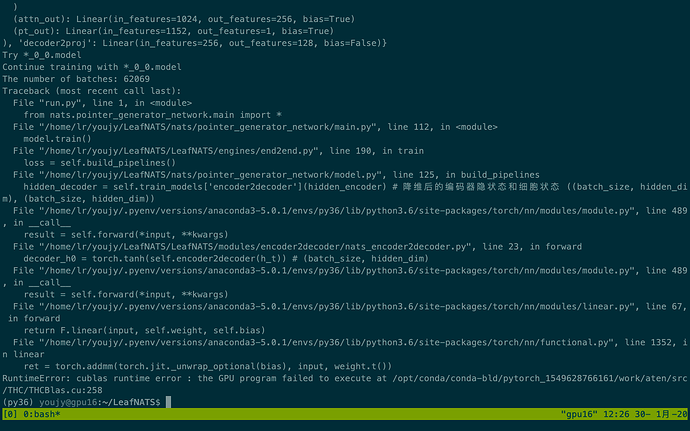# Hi everyone, I tried to run the pointnet module on Pytorch but got the following error:11440×900 132 KB

I tried to search on the Internet but cannot find any posts that are helpful.

======================
Here is the versions installed:
GPU info: GeForce RTX 2080
\$ nvidia-smi
It shows “Driver Version: 418.67, CUDA version: 10.1”

\$nvcc -V
It shows “Cuda compilation tools, release 10.1, V10.1.168”

Pytorch version: 1.0.1.post2 (installed by anaconda3)
Python version: 3.6.8

If you can give me hand I will be really thankful

Could you post a code snippet to reproduce this issue, please?

Thanks for your reply. I run this following code and the error happans

Thanks I reproduce it as following, and the error happans at
self.encoder2decoder = nn.Linear(2*src_hidden_size, trg_hidden_size)

class natsEncoder2Decoder(nn.Module):

``````def __init__(self, src_hidden_size, trg_hidden_size, rnn_network):
super(natsEncoder2Decoder, self).__init__()
self.rnn_network = rnn_network

self.encoder2decoder = nn.Linear(2*src_hidden_size, trg_hidden_size)
if rnn_network == 'lstm':
self.encoder2decoder_c = nn.Linear(2*src_hidden_size, trg_hidden_size)

def forward(self, hidden_encoder):
if self.rnn_network == 'lstm':
(src_h_t, src_c_t) = hidden_encoder
h_t = torch.cat((src_h_t[-1], src_h_t[-2]), 1)
c_t = torch.cat((src_c_t[-1], src_c_t[-2]), 1)

decoder_h0 = torch.tanh(self.encoder2decoder(h_t)) # (batch_size, hidden_dim)
decoder_c0 = torch.tanh(self.encoder2decoder_c(c_t))

return (decoder_h0, decoder_c0)

else:
src_h_t = hidden_encoder
h_t = torch.cat((src_h_t[-1], src_h_t[-2]), 1)

decoder_h0 = torch.tanh(self.encoder2decoder(h_t))

return decoder_h0``````